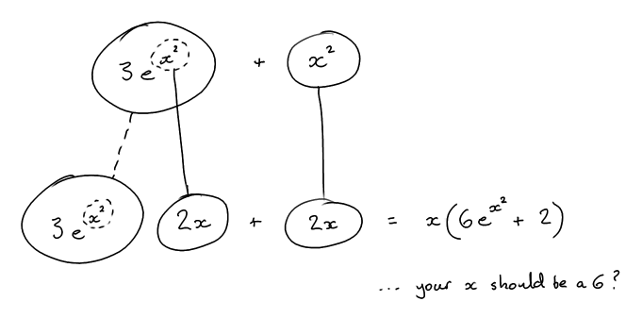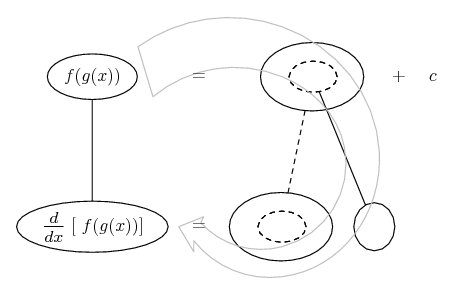# Thread: Help in Differentiating :) Exam tomorrow so please help!! :-D

1. ## Help in Differentiating :) Exam tomorrow so please help!! :-D

Hi there i need to show that the the deferential of
y=(3e^x^2) + (x^2)

is equal to

x(xe^x^2 +2)

Thanks! i have an exam tomorrow so if you could be rly kind, please help meVidhu

2. ## Re: Help in Differentiating :) Exam tomorrow so please help!! :-D

Just in case a picture helps...... where (key in spoiler) ...

Spoiler:... is the chain rule. Straight continuous lines differentiate downwards (integrate up) with respect to the main variable (in this case x), and the straight dashed line similarly but with respect to the dashed balloon expression (the inner function of the composite which is subject to the chain rule).

The general drift is..._________________________________________

Don't integrate - balloontegrate!

Balloon Calculus; standard integrals, derivatives and methods

Balloon Calculus Drawing with LaTeX and Asymptote!

3. ## Re: Help in Differentiating :) Exam tomorrow so please help!! :-D

I presume you mean 3e^(x^2) rather than, say, 3(e^x)^2 since that would be written 3e^(2x). Please use parentheses!

However, you cannot prove that the derivative of 3e^(x^2)+ x^2 is x(x e^(x^2)+ 2) because that is simply not true.

The derivative of e^u, where u is any function of x, is e^u(u'). In particular, The derivative of 3e^(x^2) is 3e^(x^2)(2x)= 6x e^(x^2). Of course, the derivative of x^2 is 2x so the derivative of 3e^(x^2)+ x^2 is 6x e^(x^2)+ 2x. You can factor out "2x" to get 2x(3e^(x^2)+ 1) which is NOT x(xe^x^2 +2).

#### Search Tags

differentiating, exam, tomorrow